top of page
Search

# Conquering Capsules

A long time ago, in the New York Times Magazine, there was a weekly puzzle called Capsules.* It was super fun. Now, the thing with Capsules is that no one's heard of them, and importantly, they're hard to find on the Internet. But Capsules has all the ingredients of success–it's simple to learn, it has a lot of depth, and it has unique theoretical ramifications.

So this post will be an introductory course to Capsules–first, the rules. Then, to reinforce the rules and explain a few useful solving techniques, I'll walk you through the solution of a Capsules puzzle.

The Rules

The goal of Capsules is to fill in the grid with a number in each square such that:

1. Each bolded cage of size 4 has the numbers 1, 2, 3, and 4; each cage of size 5 has 1, 2, 3, 4, and 5; generally, each cage of size n has numbers 1 through n.

2. No two of the same number can touch, not even diagonally.

Lez Go

Here's our starting grid:What can we get right off the bat? Well, look at the cage on the left side of the grid that I've outlined in purple. It only has one square! Since it's a cage of size 1, it has the numbers 1 through 1–that is, it has the number 1. This is known as a freebie. Let's fill it in.In this case, the freebie isn't really helping us, so let's move our attention to the next cage I've outlined in purple, the one shaped like a sideways T. (In fact, let's save some time here–whenever a cage is outlined in purple, it means that I'm going to talk about it.) Where can the 1 go in that cage? Well, no two of the same number can touch, not even diagonally. And there's a 1 touching every square in that cage except for one. So the remaining square has to be a 1, since there's nowhere else the 1 can go.Jacob's Rule

Before we move on, this principle is so important that it has a name–Jacob's rule. It has two main forms, as illustrated in the diagrams below (which are not supposed to be an entire puzzle, by the way, just a section.)

In the left example, the two highlighted squares have to be equal. Why? Because the yellow box on the left touches every other box in the cage, so no matter what's there, it only has one space to go in the other box. Illustrated is an example of when that would be useful. The left yellow box touches a 2 and a 4, so it can't be either of those, whereas the right yellow box touches a 1, 2, and 5. Normally, we couldn't go further, but Jacob's Rule tells us they are equal, so they must both be 3s. Note that if we knew beforehand the value of either box, we could use that to get the other box, like we did in the puzzle.Now let's examine the right example. The lower cage has four squares, and they all touch the highlighted square in another cage. That means that the yellow square is touched by a 1, a 2, a 3, and a 4, in some order. Therefore, that box can't be a 1, 2, 3, or 4, and since the cage it's in has five boxes, it must be a 5. Jacob's Rule comes in handy a lot, and you should always look out for it–but be careful. It works best when the sizes of the cages you're comparing are equal or only different by 1.

Back to the Main Puzzle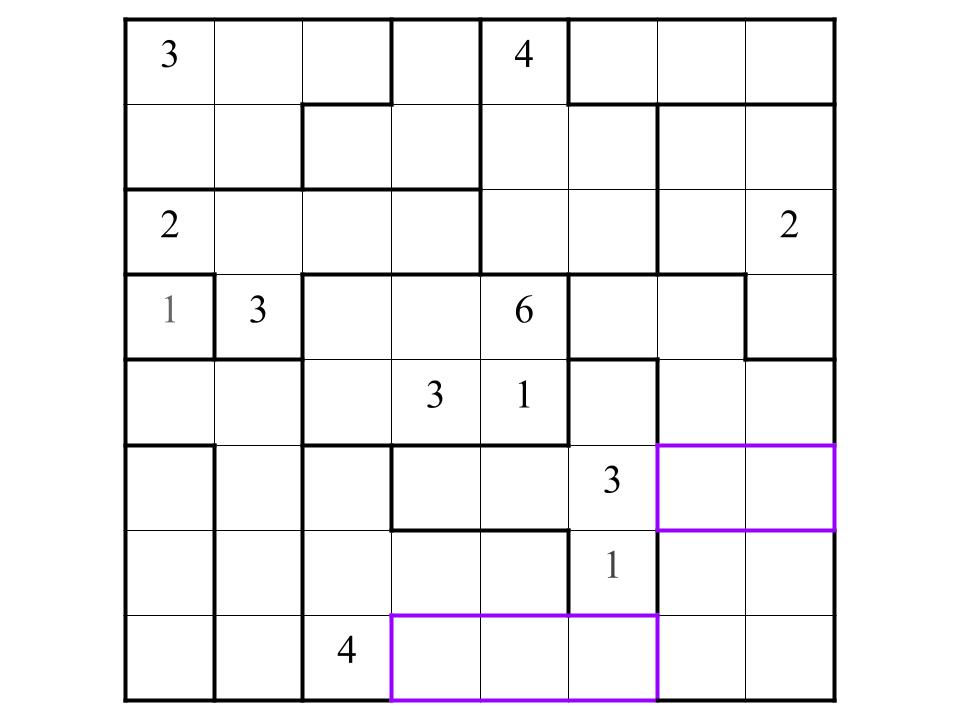As usual, examine the purple cages. In both, you'll see that the 1 has only one space to go. In the right purple cage, there are only two boxes. So the other box must be a 2.This purple-outlined cage's top left square can't be a 1, 2, or 3, so it's a 4; its top right square touches a 1 and 2, and the 4's already used, so it's a 3. The bottom two squares are just 1 and 2 then. The bottom left box touches a 1, so it's a 2, and the bottom right square is forced to be 1. In the future, I won't write all this logic out, I'll just say something like, "We now fill in the purple-outlined cage."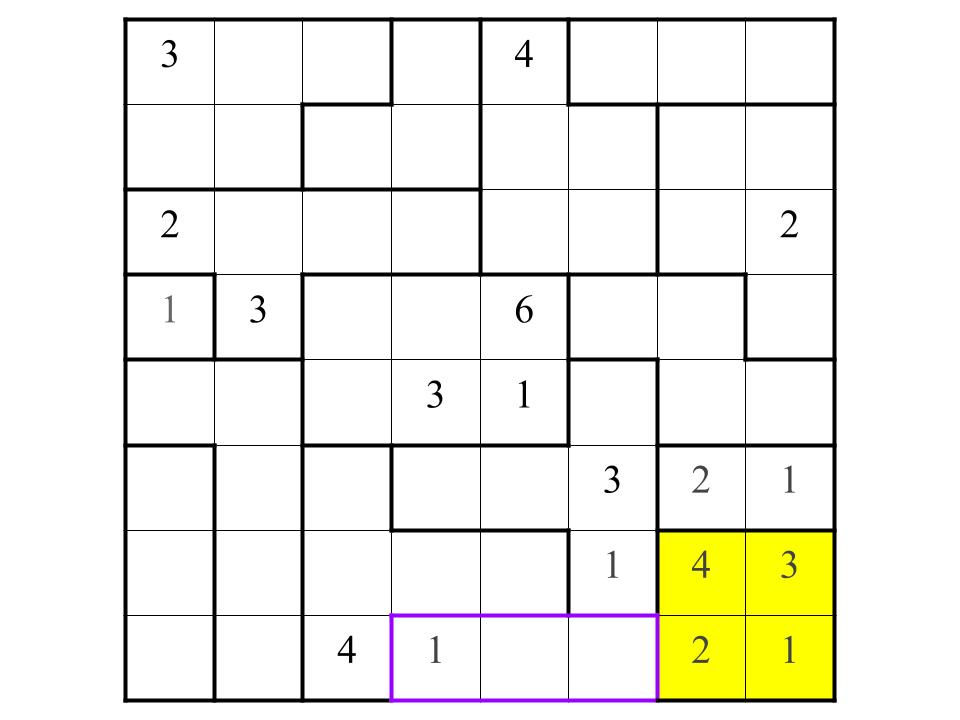The remaining numbers in the purple-outlined cage are 2 and 3, which we can now place.We can place the 1 in this cage because of Jacob's Rule. We can then fill in the rest of the cage because of the 2 and 3 below it.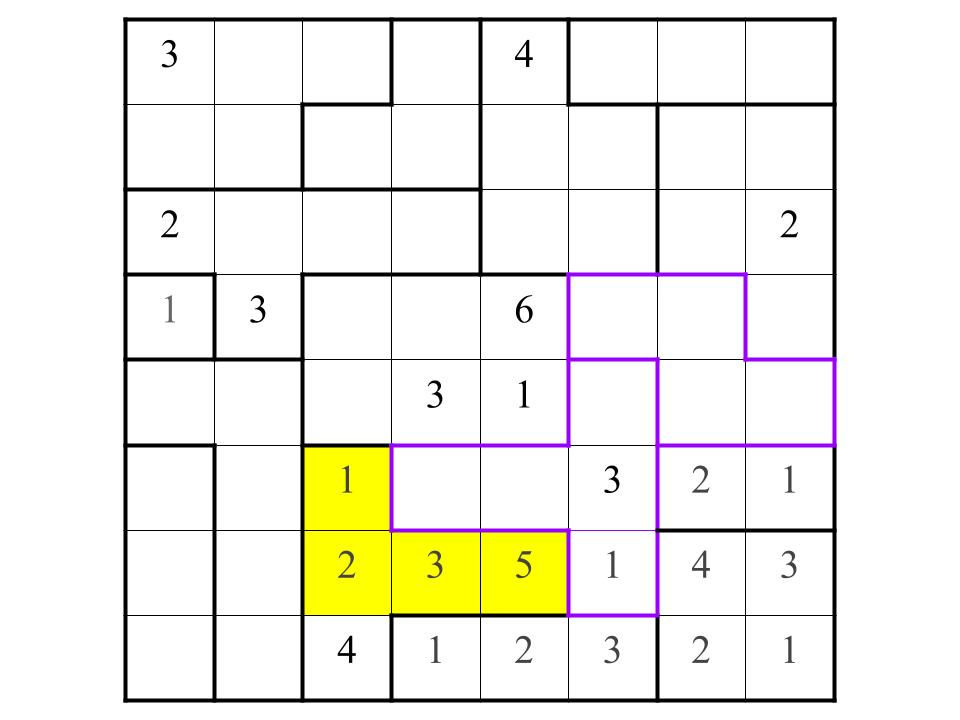We can now completely fill in two more cages.Towards the top right of the board, I've outlined in purple a single box instead of the whole cage. That's because the box tells us all we need to know. It borders a 1, 2, 3, and 4, so it has to be a 5, but we don't know anything else about the box. Meanwhile, the purple-outlined cage towards the bottom left has only one place for the 1.The top box in this cage is the only place where the 1 can be. The other two squares are a 2 and 3 in some order. Whenever you have two numbers that can go in either of two spaces, this is called a pointing pair, and it's worth notating for reasons that will become apparent soon. In fact, even if you only know one number in the pointing pair–for instance, "The 4 can go in this square or this square," it's still worth writing down if you're stuck.Within the purple-outlined cage, the box above the 1 is guaranteed to be a 5. Why? It touches a 1, 4, and our 2/3 pointing pair. See, that's why the pointing pair came in handy–because no matter where the 2 and 3 are, we can still use them to make a logical deduction.Now it's time to resolve the 2/3 situation. Which is a 2 and which is a 3? We apply a method called uniqueness. Here's the idea behind uniqueness: by definition, this puzzle has one unique solution. In other words, something has to force that 2 and that 3 in their places. In this grid, you can see that there's only one square that can do it, and it's right above the 5. Whether that square is a 2 or 3 will determine the value of our favorite pointing pair. But it borders a 2 diagonally, so it must be a 3, and so we have our answer. But wait, you might be saying. Why can't the number above the 5 be a 4? If you trust that this puzzle has a unique solution, it can't be, because then the 2 and 3 in our pointing pair can be reversed, and there will be two solutions.**

Be very careful with uniqueness, by the way. Double-check that you've found the only way a pointing pair can be resolved, because often there's a way you didn't see and you've completely messed up your puzzle.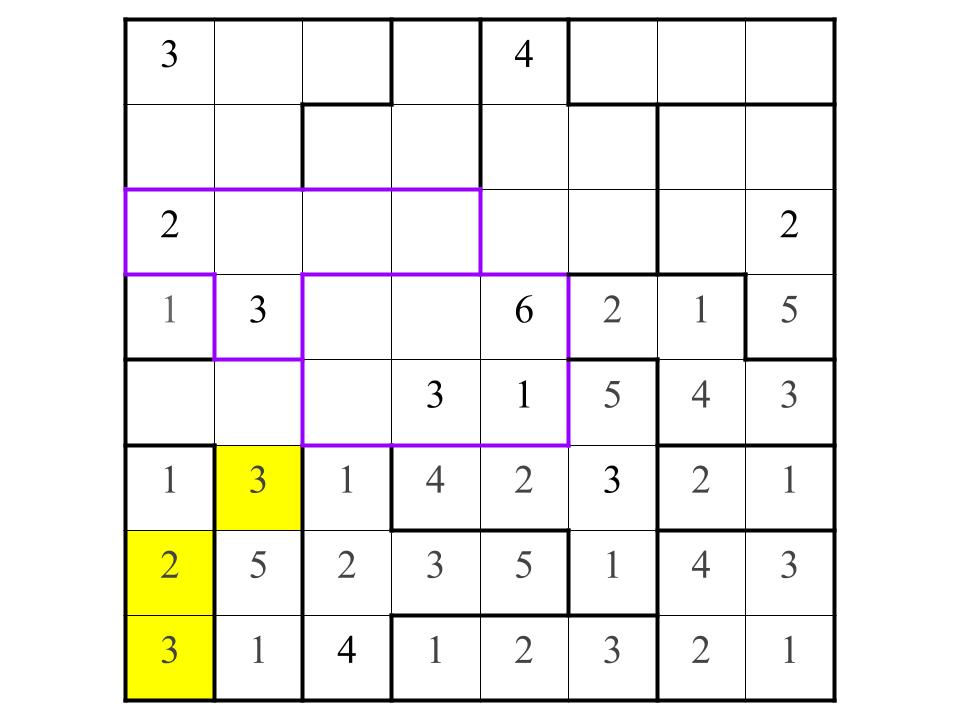The Sidestep Rule

Where can the 4 go in each of the purple-outlined cages? Well, one box in the 6-square cage touches a 4, but otherwise, it can go wherever.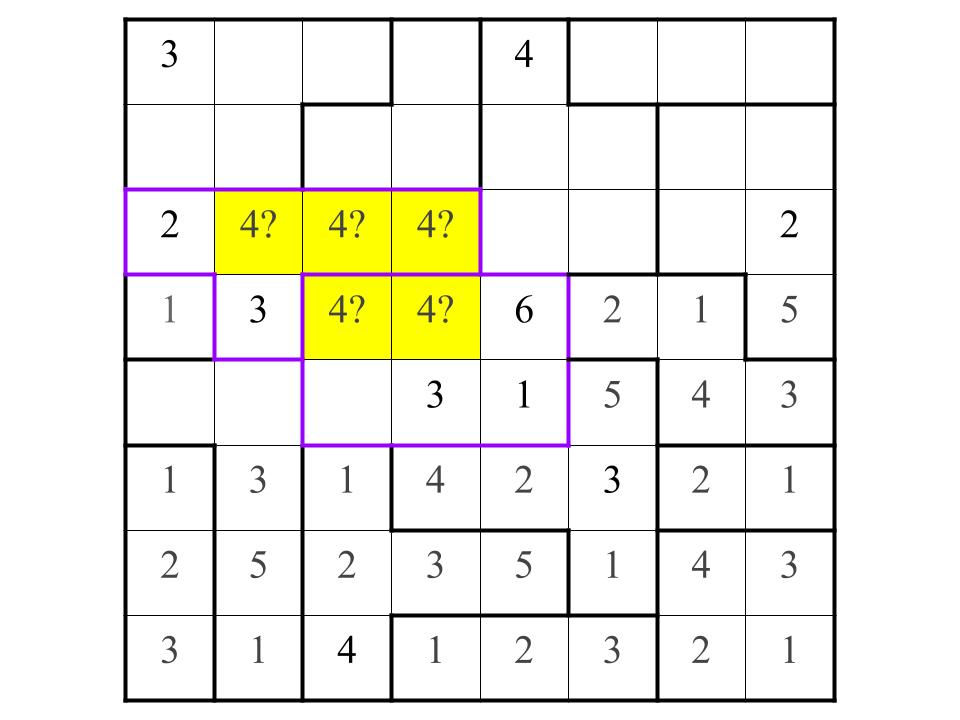This is called the Sidestep Rule because the two 4s have to sidestep each other, managing to stay in their cages while not making contact with the other 4. Fortunately, there's only one arrangement where this is possible, with the 4s at opposite extremities.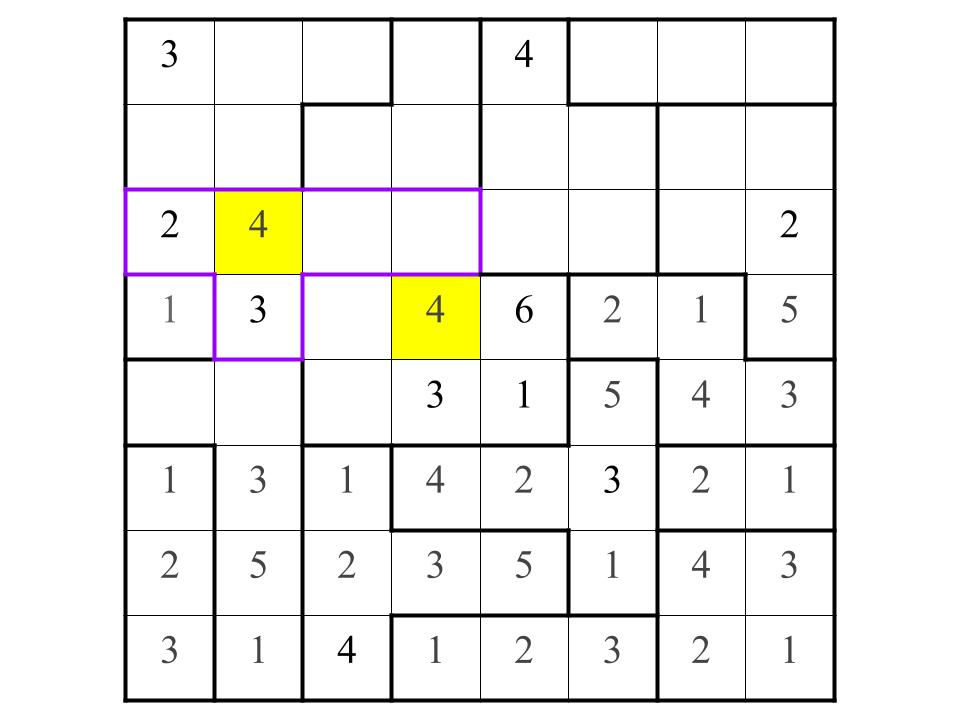Return of the Pointing Pair

The two remaining squares in the purple-outlined cage must be a 1 and a 5 respectively, so we have an additional pointing pair.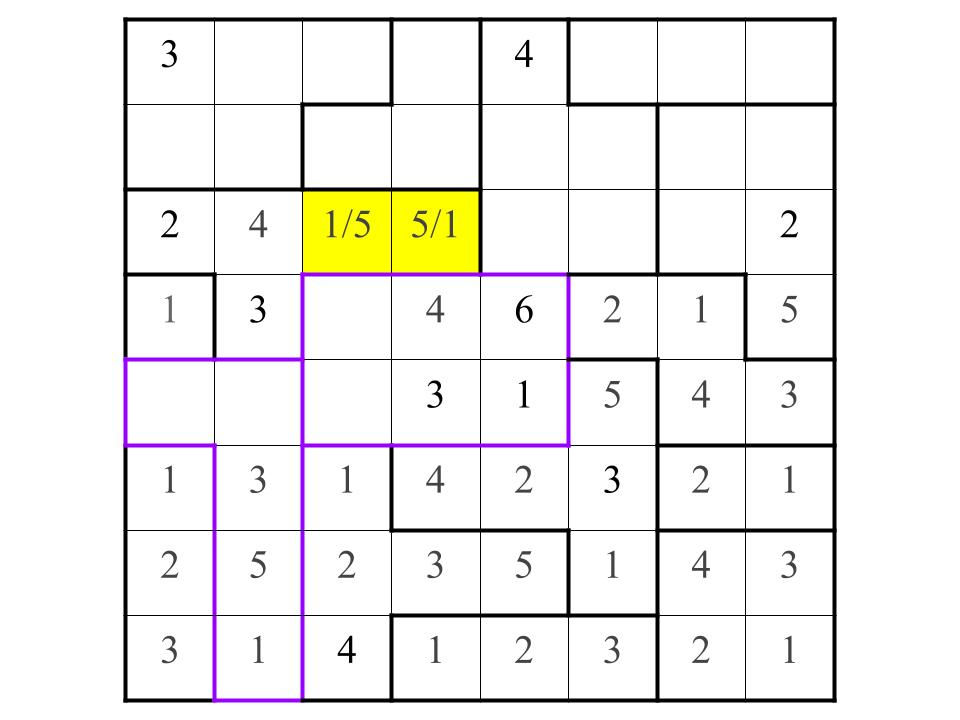The pointing pair forces the remaining numbers in the two purple-outlined cages.We turn our attention to the box outlined in purple in the top-right quadrant of the puzzle. Since the box touches every square in a cage of size 3, it's either a 4 or 5 by Jacob's Rule. However, it shares a cage with a 5. That forces it to be a 4.That tells us the numbers in the rest of the cage, along with the 1 in the cage above it.We can now completely finish the two cages outlined in purple.That resolves the pointing pair question.The Rest is Trivial

And then finally......we are done!

Finding Capsules Puzzles on the Internet

I hope that has given you an appreciation for the intriguing logic required to solve a capsules puzzle. But, more realistically, you have nothing better to do. If that's the case, I've linked some puzzles below, of my own creation, that I recommend you print out and solve for yourself, in order of difficulty:

"Columns" (Easy)

"Nueva" (Easy)

"Nesting" (Medium)

"Normalcy" (Medium)

"Rotation" (Hard)

"Nine Percent" (Hard)

Once you're done with those, there is exactly one place I've found them on the Internet, where they're made by the Computer Science Department of WPI college. In these 10x10 puzzles, all cages have exactly 5 squares. They're generated by a computer algorithm, which creates a valid solution to the puzzle, and then decides which squares will be givens. Then, the computer randomly solves the puzzle many times. If it gets the same answer every time, it determines that the puzzle has a unique solution, and is done with it.

Since these puzzles aren't created or edited by humans, they're not particularly elegant, and often have uneven difficulty. But what they lack in quality, they make up for in quantity. I'm immensely grateful for all the fun solving experiences I've had with these puzzles. Note that each puzzle has a filename of the form Capsules-[Number of Givens]-[Random Number]. For instance, if a puzzle was Capsules-30-108, it would have 30 givens. The 108 is irrelevant. By the way, 30 givens is probably a decent place to start. You can adjust the number depending on how difficult you find that to be.

[Edit from the future: Capsules puzzles can be found on the internet if you just search under their more common name–Suguru. Here's a link to a nearly-unlimited collection of puzzles.]

Happy puzzling!

–beautifulthorns

*It was replaced by Nurikabe, which was replaced by Islands, which was replaced by Walls, which was replaced by Triple Treasure, which is the current puzzle in that slot. Of course, that's my memory, so it's probably missing some puzzles in between.

**We could also have noticed that the 3 has only one spot to go in the purple-outlined box, put it there, and then we're done with the pointing pair question. But there aren't many situations where you can apply uniqueness, and I wanted to show it off.

130 views

See All
bottom of page